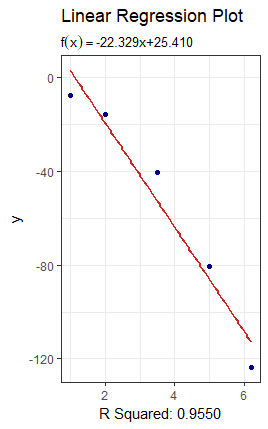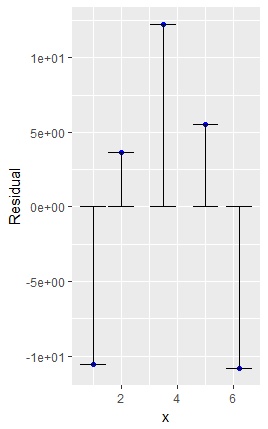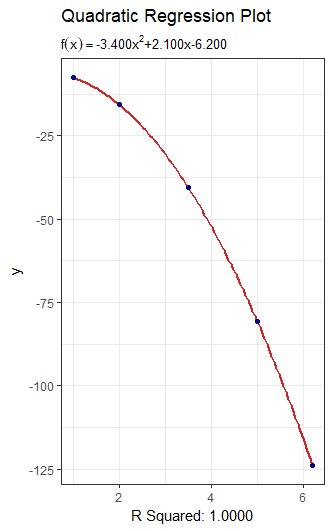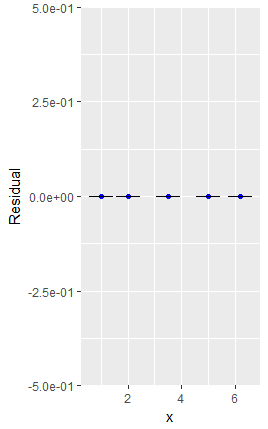Enter your search above to see Results

Whitepapers

# Polynomial Regression

The theory, math and how to calculate polynomial regression.

## An Algorithm for Polynomial Regression

We wish to find a polynomial function that gives the best fit to a sample of data. We will consider polynomials of degree n, where n is in the range of 1 to 5. In other words we will develop techniques that fit linear, quadratic, cubic, quartic and quintic regressions.  In fact, this technique will work for any order polynomial.

$f(x)=\alpha_0+\alpha_1x+\alpha_2x^2+\alpha_3x^3+…+\alpha_nx^n$ where $1\le n\le5$

The function will only approximate the value that we have in our sample data and consequently there will be a residual error $e$.

$y_i=f(x_i)+e_i$

Let us consider the set of sample observations $(x_i, y_i)$ where $i \in I$.

For each observation $i \in I$ we will have an equation:

$y_1=\alpha_0+\alpha_1x_1+\alpha_2x^2_1+\alpha_3x^3_1+…+\alpha_nx^n_1+e_1$

$y_2=\alpha_0+\alpha_2x_1+\alpha_2x^2_2+\alpha_3x^3_2+…+\alpha_nx^n_2+e_2$

$y_i=\alpha_0+\alpha_1x_i+\alpha_2x^2_i+\alpha_3x^3_i+…+\alpha_nx^n_i+e_i$

As a matrix equation polynomial regression becomes:

$\begin{bmatrix}y_1\\y_2 \\ \vdots \\y_i\end{bmatrix} = \begin{bmatrix}1 & x_1 & x_1^2 & x_1^3 \dots x_1^n \\ 1 & x_2 & x_2^2 & x_2^3 \dots x_2^n \\ \vdots & \vdots & \vdots & \vdots \\1 & x_i & x_i^2 & x_i^3 \dots x_i^n \end{bmatrix}\begin{bmatrix} \alpha_0 \\ \alpha_1 \\ \vdots \\ \alpha_i\end{bmatrix}+\begin{bmatrix} e_1\\e_2\\ \vdots \\e_i\end{bmatrix}$

Or more conveniently as:

$\hat y = M \hat \alpha + \hat e$

The residual error should be a small, randomly distributed term that we seek to minimize. Because of this we will solve the equation by setting $\hat e=0$ and solving for $\hat \alpha$.

#### Squaring the Matrix

The matrix $M$ has $i$ rows and $n+1$ columns and so is probably not square because we usually have many more observations than the degree of the polynomial. This means that we can’t calculate an inverse of $M$ because only square matrices can be inverted.

The trick we use here is to multiply the equation by the transposition of $M$ which we will denote by $M’$. When we transpose a matrix we simply swap its rows and columns.

Firstly, let us multiply our equation on both sides by $M’$ to give us $M’ \hat y=M’M \hat\alpha$

Let us think about the dimensions of the last equation. The matrix $M$ has dimensions $\{i,n+1\}$ so the matrix $M’$ must have dimensions $\{n+1,i\}$ and the product $M’M$ will have dimensions $\{n+1,n+1\}$ and so is forced to be a square matrix.  The size of the matrix depends on the polynomial we wish to fit.  For example if we are fitting a quadratic regression it will be a $\{3,3\}$ or three-by-three matrix.  $\hat y$ and $\hat \alpha$ are vectors with $i$ members so have dimension $\{i,1\}$.  We can now see the dimensionality of the full equation:

$\{n+1,i\}\{i,1\}=\{n+1,i\}\{i,n+1\}\{i,1\}$

$\{n+1,1\}=\{n+1,n+1\}\{i,1\}$

$\{n+1,1\}=\{n+1,1\}$

So both sides of the equation end up as vectors with n+1 numbers.  This means we have a series of n+1 linear equations from which we can derive $\hat \alpha$. This obviously makes sense as to fit a polynomial regression of degree n we have n coefficients of x plus a constant value, which contributes the $+1$.

#### Solving the Equation

$M’M$ is square so we can invert it.  Consequently we multiply our equation by the inverse of $M’M$ which we show as $(M’M)^{-1}$.

$(M’M)^{-1}(M’\hat y)=(M’M)^{-1}(M’M)\hat \alpha$ but $(M’M)^{-1}(M’M)$ is simply the identity matrix so the right side is just $\hat \alpha$.

$\hat \alpha = (M’M)^{-1}(M’\hat y)$

We can look closer at the two main terms of this last equation:

$M’M=\begin{bmatrix} 1 & 1 & 1 \dots 1\\x_1 & x_2 & x_3 \dots x_i \\x_1^2 & x_2^2 & x_3^2 \dots x_i^2 \\ \vdots & \vdots & \vdots \\x_1^n & x_2^n & x_3^n \dots x_i^n \\ \end{bmatrix}\begin{bmatrix}1 & x_1 & x_1^2 & x_1^3 \dots x_1^n\\ 1 & x_2 & x_2^2 & x_2^3 \dots x_2^n \\ \vdots & \vdots & \vdots & \vdots \\1 & x_i & ;x_i^2 & x_i^3 \dots x_i^n \end{bmatrix}$

$M’M=\begin{bmatrix} I & \Sigma x_i & \Sigma x_i^2\dots\Sigma x_i^n\\ \Sigma x_i & \Sigma x_i^2 & \Sigma x_i^3\dots \Sigma x_i^{n+1}\\\Sigma x_i^2 & \Sigma x_i^3 & \Sigma x_i^4 \dots\Sigma x_i^{n+2}\\\vdots & \vdots & \vdots\\\Sigma x_i^n & \Sigma x_i^{n+1} & \Sigma x_i^{n+2}\dots \Sigma x_i^{2n}\end{bmatrix}$

Similarly $M’\hat y=\begin{bmatrix}\Sigma y_i\\\Sigma x_iy_i\\\Sigma x_i^2y_i\\\vdots\\\Sigma x_i^ny_i\end{bmatrix}$

Given our input data $\hat x$ and $\hat y$ we can easily calculate and fill these matrices and complete the equation.

## Worked Example

The above theory is quite hard to follow so we can show an easy worked example to illustrate how the numbers all work together.

Firstly we need to have some observations. We have 5 observations and we can fit a linear regression:

 $x=\{1.0,2.0,3.5,5.0,6.2\}$ $\Sigma x = 17.7$ $y=\{-7.500,-15.600,-40.500,-80.700,-123.876\}$ $\Sigma y = 268.176$ $x^2=\{1.00,4.00,12.25,25.00,38.44\}$ $\Sigma x^2=80.69$ $xy=\{-7.5,-31.2,-141.75,-403.5,-768.0312\}$ $\Sigma xy=-1351.981$

$\hat \alpha = (M’M)^{-1}(M’\hat y)$

$M’M=\begin{bmatrix} i & \Sigma x_i \\ \Sigma x_i & \Sigma x_i^2 \end{bmatrix}=\begin{bmatrix} 5 & 17.7 \\ 17.7 & 80.69 \end{bmatrix}$

$M’\hat y= \begin{bmatrix}\Sigma y_i\\\Sigma x_iy_i\end{bmatrix}=\begin{bmatrix}268.176\\-1351.981\end{bmatrix}$

$\hat \alpha=\begin{bmatrix} 5 & 17.7 \\ 17.7 & 80.69 \end{bmatrix}^{-1} \begin{bmatrix}268.176\\-1351.981\end{bmatrix}$

$\hat \alpha=\begin{bmatrix} 0.8949645 & -0.19631766\\-0.19631766 & 0.05545697 \end{bmatrix} \begin{bmatrix}268.176\\-1351.981\end{bmatrix}=\begin{bmatrix}25.40974\\-22.32908\end{bmatrix}$
So this suggests that the function $y = 25.41-22.33x$ would be a good linear regression for the data. We can obviously see if this worked by plotting our observations on a chart as blue dots and the function as a red line. This is what we see when we do this.We can clearly see that the fit looks quite good, However, if we repeat the analysis again but we try to fit a quadratic regression we get this.

$M’M=\begin{bmatrix} i&\Sigma x_i & \Sigma x_i^2\\ \Sigma x_i &\Sigma x_i^2 & \Sigma x_i^3 \\ \Sigma x_i^2 & \Sigma x_i^3 & \Sigma x_i^4 \end{bmatrix}=\begin{bmatrix} 5 & 17.7 & 80.69\\ 17.7 & 80.69 & 415.203 \\ 80.69 & 415.203 & 2269.696\end{bmatrix}$

$M’\hat y= \begin{bmatrix}\Sigma y_i\\\Sigma x_iy_i \\ \Sigma x_i^2y_i\end{bmatrix}=\begin{bmatrix}268.176\\-1351.981\\-7345.318\end{bmatrix}$

$\hat \alpha=\begin{bmatrix} 5 & 17.7 & 80.69\\ 17.7 & 80.69 & 415.203 \\ 80.69 & 415.203&2269.696\end{bmatrix}^{-1} \begin{bmatrix}268.176\\-1351.981\\-7345.318\end{bmatrix}$

$\hat \alpha=\begin{bmatrix} 3.2548847 & -2.0201426 & 0.25383691\\-2.0201426 & 1.4649696 & -0.19617361 \\ 0.2538369 & -0.1961736 & 0.02730312\end{bmatrix} \begin{bmatrix}268.176\\-1351.981\\-7345.318\end{bmatrix}= \begin{bmatrix} -6.2\\2.1\\-3.4\end{bmatrix}$

The charts now look like this:Clearly the fitted curve is now a perfect fit as the residuals are all zero and the $r^2$ is one.

#### Final Comment

The technique that we outlined here is simple and it works.  However, as we did the worked examples we started to see some of the problems in this technique.  The matrices are filled with powers and so the numbers start to get high.  If you look at the final multiplication we have the inverse matrix with small numbers multiplied by a vector with big numbers and the result has reasonable sized numbers.  This is where this technique has a problem.  When a computer runs through the math there can be issues with overflow and propagation of errors.

Enquiry